# How to Calculate and Solve for Cumulative GOR of Reservoir Fluid Flow | The Calculator Encyclopedia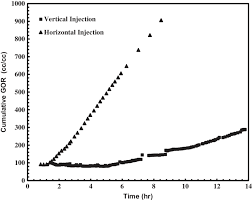The image above represents the cumulative GOR.

To compute for the cumulative GOR, two essential parameters are needed and these parameters are cumulative gas production (Gp) and cumulative oil production (Np).

The formula for calculating the cumulative GOR:

Rp = Gp / Np

Where;

Rp = Cumulative GOR
Gp = Cumulative Gas Production
Np = Cumulative Oil Production

Let’s solve an example;
Find the cumulative GOR with a cumulative gas production of 32 and a cumulative oil production of 44.

This implies that;

Gp = Cumulative Gas Production = 32
Np = Cumulative Oil Production = 44

Rp = Gp / Np
Rp = 32 / 44
Rp = 0.72

Therefore, the cumulative GOR is 0.72.

Calculating the Cumulative Gas Production when the Cumulative GOR and the Cumulative Oil Production is Given

Gp = Rp x Np

Where;

Gp = Cumulative Gas Production
Rp = Cumulative GOR
Np = Cumulative Oil Production

Let’s solve an example;
Find the cumulative gas production when the cumulative GOR is 56 and the cumulative oil production is 19.

This implies that;

Rp = Cumulative GOR = 56
Np = Cumulative Oil Production = 19

Gp = Rp x Np
Gp = 56 x 19
Gp = 1064

Therefore, the cumulative gas production is 1064.

Calculating the Cumulative Oil Production when the Cumulative GOR and the Cumulative Gas Production is Given

Np = Gp / Rp

Where;

Np = Cumulative Oil Production
Rp = Cumulative GOR
Gp = Cumulative Gas Production

Let’s solve an example;
Find the cumulative oil production when the cumulative GOR is 18 and the cumulative gas production is 72.

This implies that;

Rp = Cumulative GOR = 18
Gp = Cumulative Gas Production = 72

Np = Gp / Rp
Np = 72 / 18
Np = 4

Therefore, the cumulative oil production is 4.

Nickzom Calculator – The Calculator Encyclopedia is capable of calculating the cumulative GOR.

To get the answer and workings of the cumulative GOR using the Nickzom Calculator – The Calculator Encyclopedia. First, you need to obtain the app.

You can get this app via any of these means:

To get access to the professional version via web, you need to register and subscribe for NGN 1,500 per annum to have utter access to all functionalities.
You can also try the demo version via https://www.nickzom.org/calculator

Apple (Paid) – https://itunes.apple.com/us/app/nickzom-calculator/id1331162702?mt=8
Once, you have obtained the calculator encyclopedia app, proceed to the Calculator Map, then click on Petroleum under EngineeringNow, Click on Reservoir Fluid Flow under Petroleum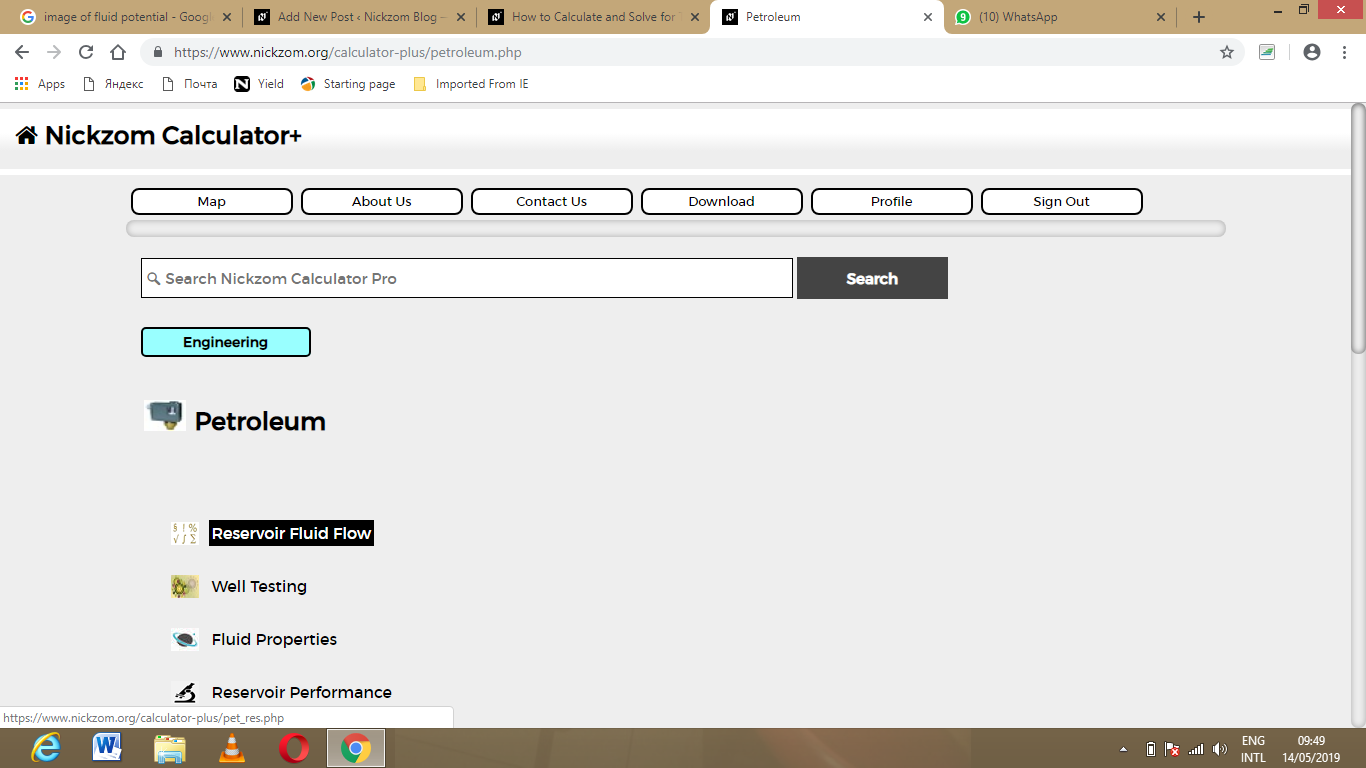Now, Click on Cumulative GOR under Reservoir Fluid Flow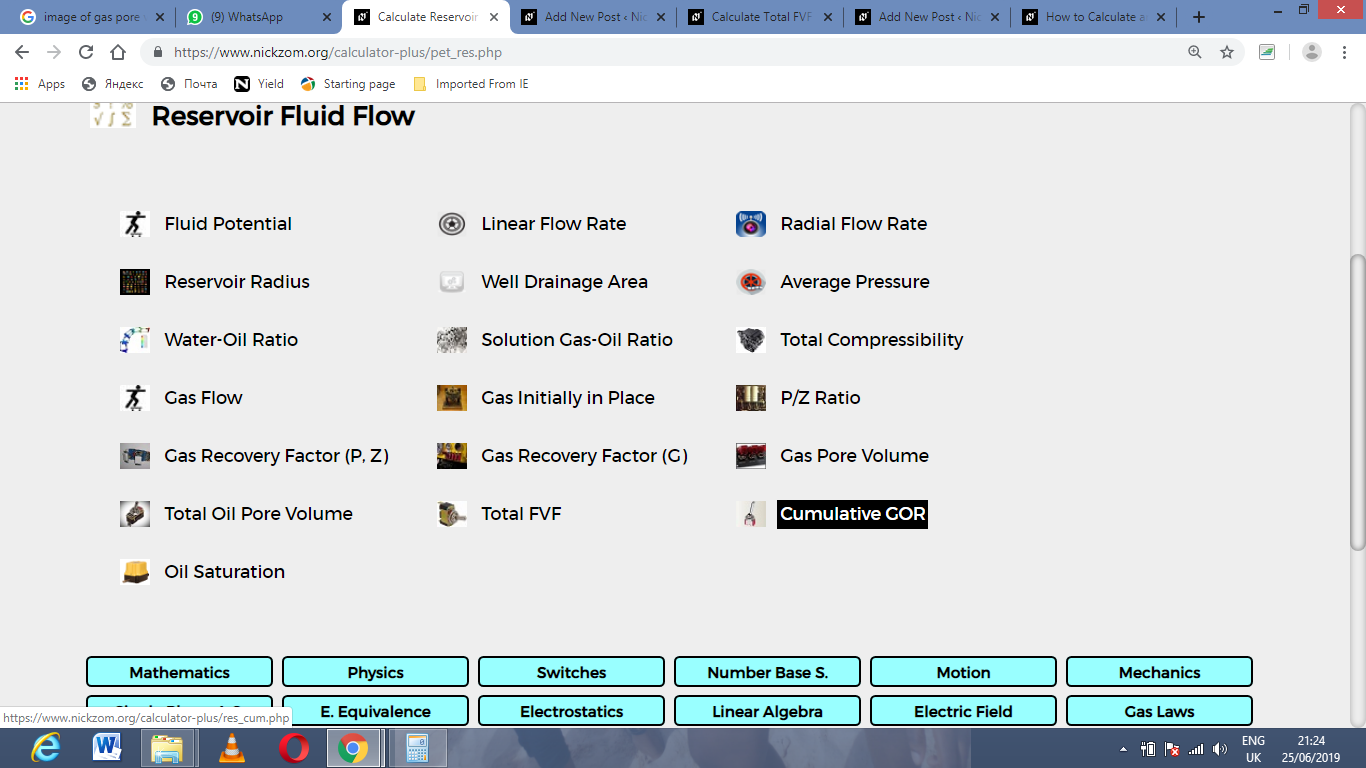The screenshot below displays the page or activity to enter your value, to get the answer for the cumulative GOR according to the respective parameter which are the cumulative gas production (Gp) and cumulative oil production (Np).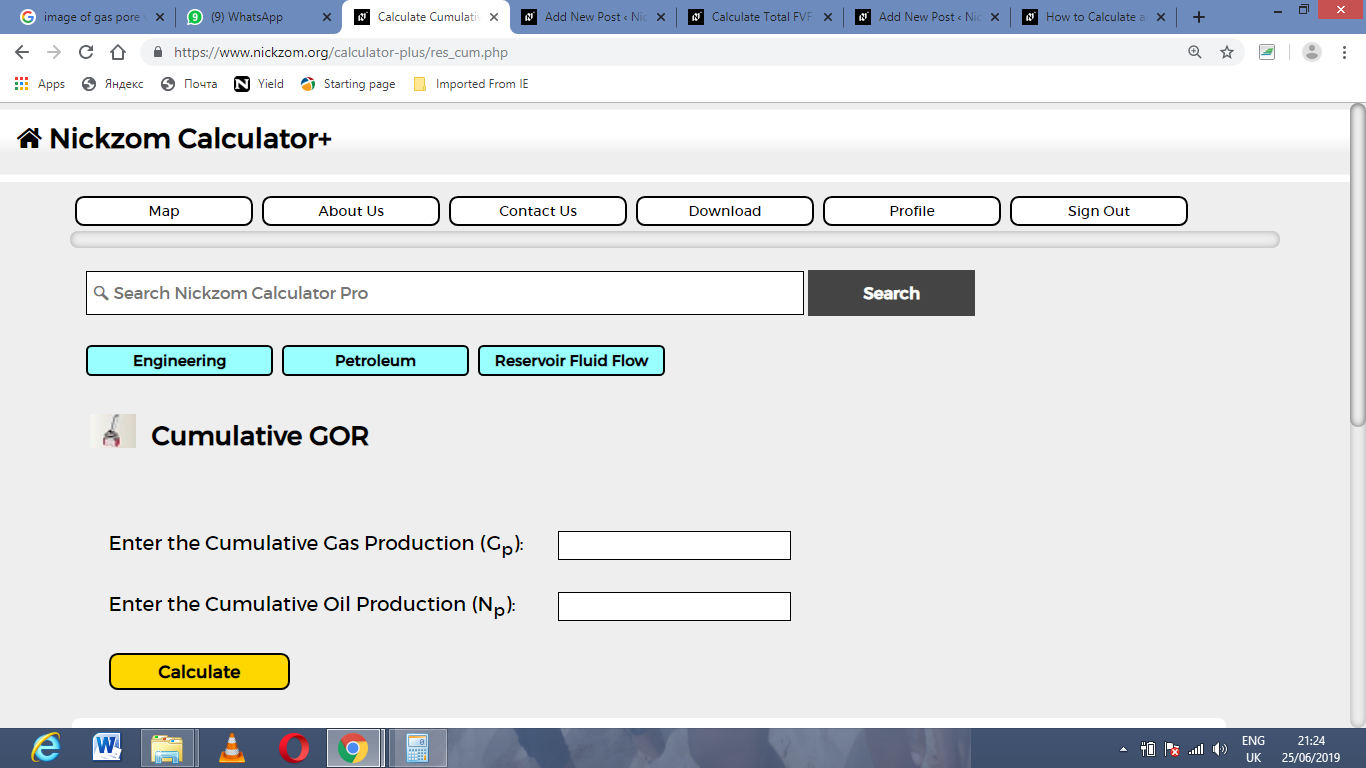Now, enter the value appropriately and accordingly for the parameter as required by the cumulative gas production (Gp) is 32 and cumulative oil production (Np) is 44.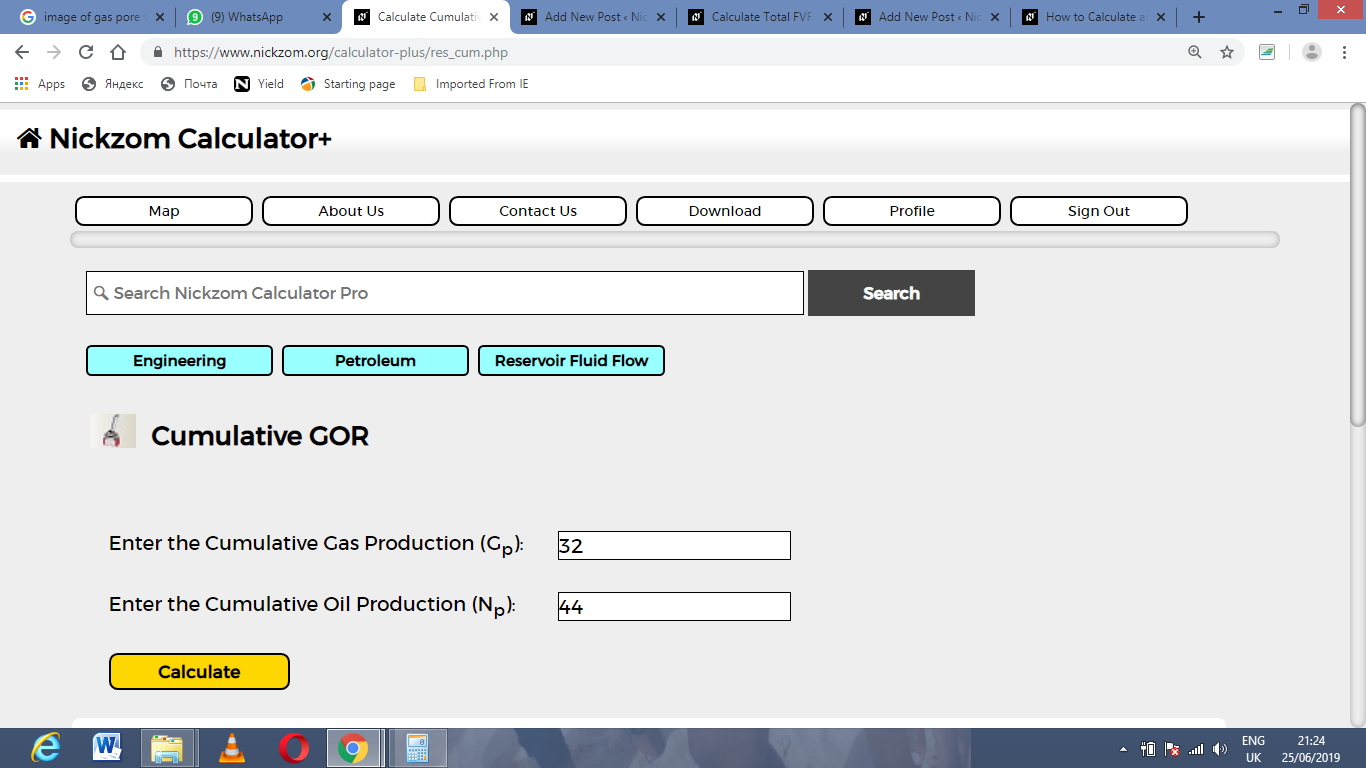Finally, Click on Calculate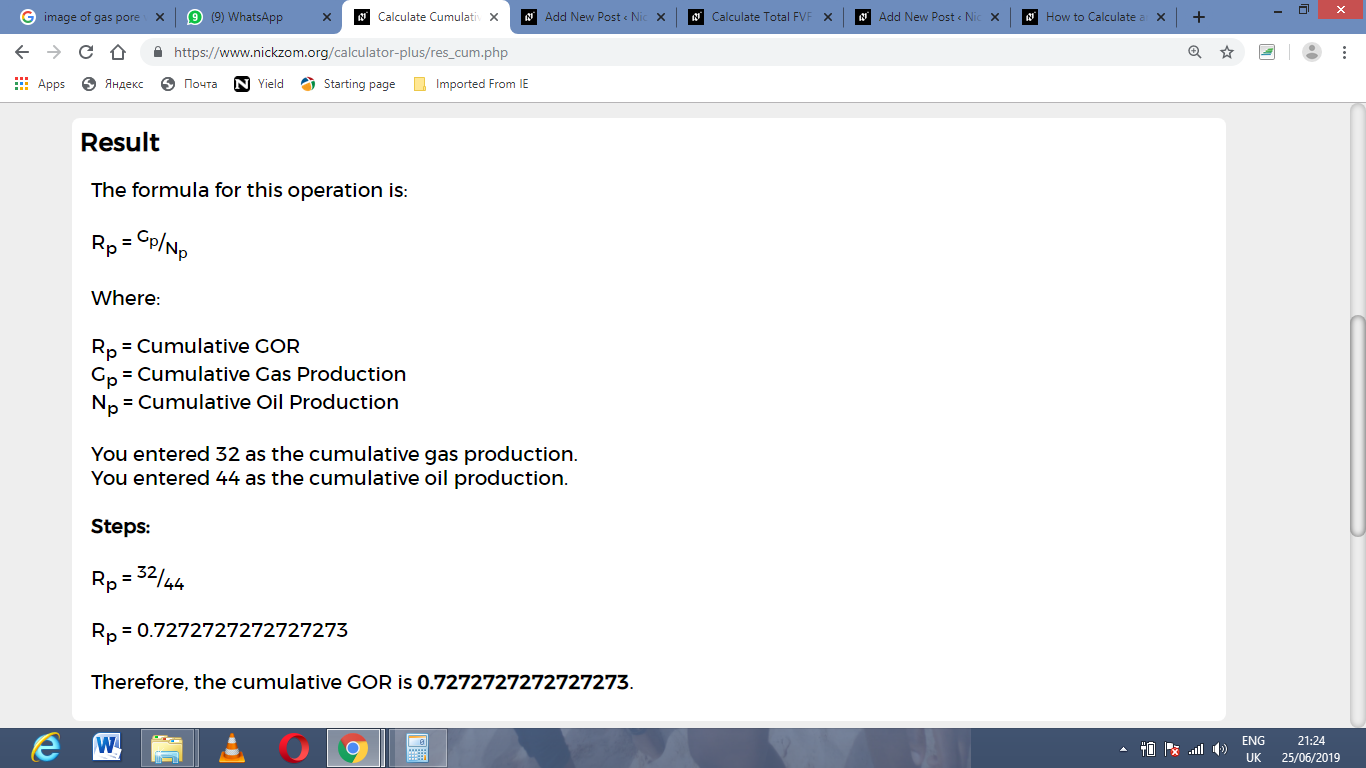As you can see from the screenshot above, Nickzom Calculator– The Calculator Encyclopedia solves for the cumulative GOR and presents the formula, workings and steps too.